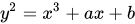I want to start today a series of posts on a fascinating topic: elliptical curves, and my personal path to learn about it. I am not going to approach the subject from the point of view of cryptography or the applications of elliptic curves. Rather, I am going to focus on the importance they have in modern mathematics. They are an element that serves to reveal the unity of mathematics, and how certain subjects that seem disconnected, instead have an unexpected relationship.

My first serious encounter with elliptic curves was in the 1990s, when Wiles’ famous proof of Fermat’s Last Theorem arrived. To begin to see how extensive and important the subject is, I recommend a quick read of the Wikipedia article on elliptic curves.

There we will find an expression:The simple form of an elliptic curve (Weierstrass equation) From Wikipedia

And that this expression depends on factors a and b. Both the x, y factors and variables can be explored by taking integer, rational, real, complex values. We could also use finite fields. In all these areas, elliptical curves have something new to tell us. It is usual to graph elliptic curves using real values:

The presence of the square of y gives to the elliptic curve a symmetry over the axe x. This is an essential step to have many interesting properties.

In this first post, I want to comment about a very interesting book that I’m reading, where the subject of elliptic curve is develope

In this post I want to comment on an excellent book that I am reading, where the topic of elliptic curves is treated from the perspective of the proof of Fermat’s Last Theorem. It is the “Algebraic number theory and Fermat’s last theorem” by Ian Stewart and David Tall. Ian Stewart is well known for his popular books on mathematics, but in this, along with Tall, he displays his clarity and didactics in explaining pure mathematical topics.

Note that this is not a book on elliptic curves. This topic occupies only a few chapters. But as a text it is very good to begin to understand the relations of these curves to various areas of mathematics. One of those places is algebraic numbers. The authors write:

The book devotes many chapters to the development of algebraic number theory. And if you don’t know anything about this subject, it’s a good introduction. All this with focus in Fermat’s Last Theorem. But at the end, this path, algebraic number theory, was not enough to get a full proof. So, the authors write:

To get to the point, what does this book about elliptic curves give us? The main development is in chapter 13, along with previous and following chapters that give perspective to the historical development. They wrote:

In that chapter, the authors shine. Instead of facing the elliptical curves DIRECTLY, they choose to explain them by going to a more familiar topic: the conics. And they extend both concepts to the projective plane, and using complexes. They show the properties of rational points in conics, and then extend them to the elliptic curves. They describe the tangent/secant process to add rational points, obtaining rational points over elliptic curves. Such operation generates an abelian group structure, being the associative law proof the decisive step in the proof. That group of rational points can be finitely generated (Mordell’s theorem). Notably, they show an application of these ideas to the solution of diophantine equations. In the following chapter, the authors show in details some relations between trigonometry and elliptic functions, using complex analysis. For example, they argue that a circle is, at last, a parametric curve, where the points x, y are functions of a parameter t, where x = sin (t), y = cos (t). The same then show for elliptic functions , a subject related historically to the elliptic curves. They can be used as parametric functions of elliptic curves, with x = f (t), y = g (t) and the function g is the derivative of f. Remarkable and not trivial fact. So, those elliptic functions are like the trigonometric functions of the circle. All these subjects, explained from the humble beginning: the circle and its properties. Then, they develop all the relation of this discoveries that paved the path to Fermat’s Last Theorem, using Taniyama-Shimura-Weil Conjecture, Frey elliptic curve, modular elliptic functions, even Galois theory.

In short, this book is a long but fascinating travel, visiting many places that seem unrelated, that sheds light on the beauty and unity of mathematics.